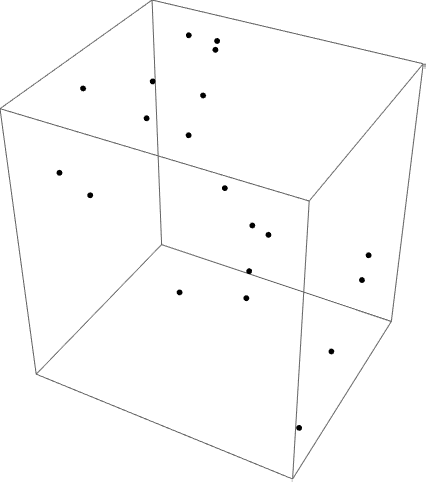# Curse of Dimensionality

In machine learning, like many fields of computer science, often involves high dimensional points, and high dimension spaces have some surprising probabilistic properties.

A random value $X_i$ is a Uni(0, 1).
A random point of dimension $d$ is a list of $d$ random values: $[X_1 \dots X_d]$.A random value $X_i$ is close to an edge if $X_i$ is less than 0.01 or $X_i$ is greater than 0.99. What is the probability that a random value is close to an edge?

Let $E$ be the event that a random value is close to an edge. $P(E) = P(X_i < 0.01) + P(X_i > 0.99) = 0.02$

A random point $[X_1, X_2, X_3]$ of dimension $3$ is close to an edge if any of its values are close to an edge. What is the probability that a $3$ dimensional point is close to an edge?

The event is equivalent to the complement of none of the dimensions of the point is close to an edge, which is: $1 - (1 - P(E))^3 = 1 - 0.98^3 \approx 0.058$

A random point $[X_1, \dots X_{100}]$ of dimension $100$ is close to an edge if any of its values are close to an edge. What is the probability that a 100 dimensional point is close to an edge?

Similarly, it is: $1 - (1 - P(E))^{100} = 1 - 0.98^{100} \approx 0.867$
There are many other phenomena of high dimensional points: such as, the euclidean distance between points starts to converge.